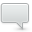Tutorials
••learning

# Sound Synthesis, Sound Design and Audio Processing - Part 3

What's the Frequency?
• Comment
• 0 comment
• Like
• Tweet
• Pin it
• Submit
• Email

As we saw in the previous article of this series, if a waveform is recurrent, it is called a periodic wave. From a theoretical point of view, for example, musical notes are all based on a periodic waveform. Noise, on the other hand, isn't, which means you cannot establish a repeating pattern for a waveform representing noise. All other sounds are somewhere between these two extremes.

## Repetition

If you recall the previous article, periodic waves follow cycles. The number of cycles that a sound completes in a second determines its oscillation rate, but not its propagation speed, which will remain the same as long as the medium – an "elastic" one, if you remember our first article in the series ─ doesn't change. The oscillation speed of a wave is expressed in Hertz (Hz), after German acoustician Heinrich Hertz, and it is called "frequency." For example, the "A" produced by a tuning fork or a sound synthesis instrument is, theoretically, made up of a simple sine wave with a frequency of 440 Hz.

The frequency value in Hz is doubled when you change octaves for the same note.

## The giant and the dwarf

To understand the difference between a wave's frequency (oscillation rate) and speed of propagation, consider a giant and a dwarf that travel the same distance ─ say, for instance, 1,125 feet ─ in the exact same time ─ one second, for example. They would both cover the 1,125 ft in one second. but while the giant would only need 20 steps to do so, the dwarf would need 20,000 steps (imagine a smurf, a really tiny one).

While the propagation speed is the same for the giant and the micro-smurf ─ namely, 1,125 ft/s ─ the number of steps they need is different: 20 steps for the giant and 20,000 for the micro-smurf.

Taking it to the audio domain, we could say that the giant is a 20 Hz wave (20 "steps" or cycles per second) and the smurf a 20,000 Hz wave (20,000 "steps" or cycles per second).

OK, I'll let you in on something, I didn't actually pick these numbers by chance. In fact, 1,125 ft/s (or 340 m/s) is the average speed of sound in air ─ although it changes with temperature! ─ and 20 Hz and 20,000 Hz (20 kHz) are, theoretically, the lower and higher frequency limits that humans can hear.

We saw that a musical note is based on a given frequency. If we retake our example, the frequency of the "A" produced by a tuning fork is 440 Hz. Nevertheless, as I wrote previously, a pure note is almost nowhere to be find in nature. Most of the time, it is played by an instrument, which our ear is capable of identifying. Yet, if our ear is capable of distinguishing a human voice, a steel drum or an oboe, it means that the waveform produced by a given source contains more sonic information that makes it identifiable, even if it always sounds as the same note to our ears, an A440, for instance.

So, in our example, 440Hz is called the root note or fundamental frequency, but there are also overtones, which provide additional information that characterizes a sound.

The root note is the one that defines the pitch of a sound. The harmonic overtones (aka harmonics or harmonic partials) that follow it are whole number multiples of the fundamental frequency. Usually, even harmonics sound better to the human ear than odd ones.

There are, however, certain elements of a sound that aren't harmonics of the root note. They are called inharmonic overtones (or inharmonic partials). The farther from the root note, the less volume the overtones have.

Together, all the elements that constitute a sound ─ its root note and harmonic and inharmonic overtones ─ are called the sound spectrum.

As we mentioned above, these additional elements define the nature of the sound produced. It is indispensable to understand this when dealing with synthesis, especially with additive and physical modeling synthesis, the latter of which actually tries to recreate real sounds. But we will look into the different types of synthesis later. The next article will be dedicated to the basic waveforms that you can find in a synth!